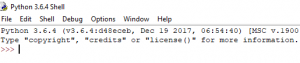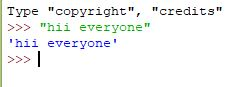# Taking input from console in Python

What is Console in Python? Console (also called Shell) is basically a command line interpreter that takes input from the user i.e one command at a time and interprets it. If it is error free then it runs the command and gives required output otherwise shows the error message. A Python Console looks like this.Here we write command and to execute the command just press enter key and your command will be interpreted.
For coding in Python you must know the basics of the console used in Python.

The primary prompt of the python console is the three greater than symbols

```>>>
```

You are free to write the next command on the shell only when after executing the first command these prompts have appeared. The Python Console accepts command in Python which you write after the prompt.Accepting Input from Console
User enters the values in the Console and that value is then used in the program as it was required.
To take input from the user we make use of a built-in function input().

 `# input ` `input1 ``=` `input``() ` ` `  `# output ` `print``(input1) `

We can also type cast this input to integer, float or string by specifying the input() function inside the type.

1. Typecasting the input to Integer: There might be conditions when you might require integer input from user/Console, the following code takes two input(integer/float) from console and typecasts them to integer then prints the sum.

 `# input ` `num1 ``=` `int``(``input``()) ` `num2 ``=` `int``(``input``()) ` ` `  `# printing the sum in integer ` `print``(num1 ``+` `num2) `

2. Typecasting the input to Float: To convert the input to float the following code will work out.

 `# input ` `num1 ``=` `float``(``input``()) ` `num2 ``=` `float``(``input``()) ` ` `  `# printing the sum in float ` `print``(num1 ``+` `num2) `

3. Typecasting the input to String: All kind of input can be converted to string type whether they are float or integer. We make use of keyword str for typecasting.

 `# input ` `string ``=` `str``(``input``()) ` ` `  `# output ` `print``(string) `

My Personal Notes arrow_drop_upCheck out this Author's contributed articles.

If you like GeeksforGeeks and would like to contribute, you can also write an article using contribute.geeksforgeeks.org or mail your article to contribute@geeksforgeeks.org. See your article appearing on the GeeksforGeeks main page and help other Geeks.

Please Improve this article if you find anything incorrect by clicking on the "Improve Article" button below.